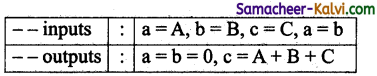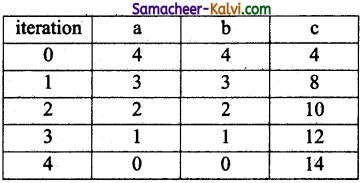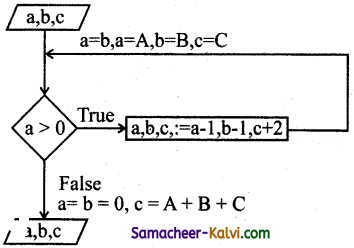## TN State Board 12th Computer Science Important Questions Chapter 1 Function

Question 1.
Define Parameters.
Parameters are the variables if a function definition mid arguments are the values which are passed to a function definition.

Question 2.
What is a recursive function?
A function definition which call itself is called recursive function.Question 3.
How will you define function?
The syntax for function definitions:
let rec fn a<sub>1</sub> a<sub>2</sub> ……….. a<sub>n</sub>: = k
Here the fn is a variable indicating an identifier being used as a function name.
The names a<sub>1</sub> to a<sub>n</sub> are variables indicating the identifiers used as parameters.
The keyword rec is required if fn is to be a recursive function, otherwise it may be omitted.

Question 4.
Write the syntax for function types.
The syntax for function types:
x → y
x<sub>1</sub> → x<sub>2</sub> → y
x<sub>1</sub> → …… x<sub>n</sub> → y
Here, x and y are variable indicating types. The type x→y is the type of a function that gets an input of type ‘x’ and returns an output of the type y.

Whereas x<sub>1</sub>→x<sub>2</sub>→y is a type of a function that takes two inputs, the first input is of type X! and the second input of type x<sub>2</sub>, and returns an output of type y.

Such as x<sub>1</sub>→ …….x<sub>n</sub>→y has type x as input of n arguments and y type as output.Question 5.
How will you solve, chameleons of chromeland problem using function?
(i) Let us represent the number of chameleons of each type by variable a,b and c, and their initial values by A,B and C, respectively.
(ii) Let a – b be the input property.
(iii) The input – output relation is a = b = 0 and c = A + B + C.
(iv) The algorithm can be specified as monochromatize (a,b,c).(vi) In each iterative step, two chameleons of the two types(equal in number) meet and change their colors to the third one. For example, if A, B, C = 4, 4, 6, then the series of meeting will result in:Question 6.
Write a monochromatize (a, b, c) algorithm and draw a flow chart.
Let A and B each decreases by a, and c increases by 2.
The solution can be expressed as an iterative algorithm.
monochromatize (a, b, c)
– – inputs: a = A, b = B, c = C, a = b
– – outputs: a = b = 0, c = A + B + C while a > 0
a, b, c: = a – 1, b – 1,c + 2.
The algorithm is depicted in the flowchart as:Question 7.
What is a subroutine?
Subroutines are the basic building blocks of computer programs.
Subroutines are small sections of code that are used to perform a particular task that can be used repeatedly.

Question 8.
Define Function with respect to Programming language.
A function is a unit of code that is often defined within a greater code structure.
A function contains a set of code that works on many kinds of inputs, like variants, expressions and produces a concrete output.Question 9.
Write the inference you get from X:=(78).
X:= (78) has an expression in it but (78) is not itself an expression.
In this case the value 78 being bound to the name X. That is definitions bind values to names.

Question 10.
Differentiate interface and implementation.

 Interface Implementation Interface just defines what an object can do, but won’t actually do it. Implementation carries out the instructions defined in the interface.Question 11.
Which of the following is a normal function definition and which is recursive function definition.
(i) let rec sum x y:
return x + y
Recursive function

(ii) let disp:
print ‘welcome’
Normal function

(iii) let rec sum num:
if (num!=0) then return num + sum (num-1)
else
return num
Recursive function

Question 12.
Mention the characteristics of Interface.

1. The class template specifies the interfaces to enable an object to be created and operated properly.
2. An object’s attributes and behaviour is controlled by sending functions to the object.
Eg: Let’s take the example of increasing a car’s speed.Question 13.
Why strlen is called Pure function?

1. Strlen is called pure function because the function takes one variable as a parameter, and accessed it to find its length.
2. This function reads external memory but does not change it, and the value returned derives from the external memory accessed.
Eg: strlen(s) is compiled, strlen needs to iterate over the whole of s.
If the compiler is smart enough to work out that strlen is a Pure function.

Question 14.
What is the side effect of impure function? Give example.
(i) Impure function has side effects when it has observable interaction with the outside world.
(ii) There are situations our functions can become impure though our goal is to make our functions pure.
(iii) Eg:
let Y: = 0
(int) inc (int)x
Y: = y + x ;
return(y)
The side effect of the inc() function is, it is changing the data 0 if the external visible variable Y.Question 15.
Differentiate pure and impure function.

 Pure Function Impure Function The return value of the pure functions solely depends on its arguments passed. Hence, if you call the pure functions with the same set of arguments, you will always get the same return values. They do not have any side effects. The return value of the impure functions does not solely depend on its arguments passed. Hence, if you call the impure functions with the same set of arguments, you might get the different return values. For example, random(), Date(). They do not modify the arguments which are passed to them. They may modify the arguments which are passed to them.

Question 16.
What happens if you modify a variable outside the function? Give an example.
Modify variable outside a function due to which the result will change, each time of the function definition.
Eg:
let x: = 0
(int) inc (int) y
x: = x + y;
return (x)
In the above example the side effect of theinc () function is, it is changing the data of the external visible variable x.Question 17.
What are called Parameters and write a note on
(i) Parameter without Type
(ii) Parameter with Type.
Parameters are the variables in a function definition and arguments are the values which are passed to a function definition.
(i) Parameter without type:
(a) In this function definition let rec pow a b: = variable b is the parameter and the value which is passed to the variable b is the argument.
(b) Here, we have not mentioned any data types.
(c) Some language compiler solves this type inference problem algorithmically.

(ii) Parameter with type:
(a) Let rec pow (a: int) (b: int): int: = in this definition for a and b the parentheses are mandatory.
(b) In this types can help with debugging such an error message.
(c) There are number of times we may want to explicitly write down types.

Question 18.
Identify in the following program
let rec gcd a b :=
if b <> 0 then gcd b (a mod b) else return a

(i) Name of the function.
gcd

(ii) Identify the statement which tells it is a recursive function.
rec

(iii) Name of the argument variable.
a, b

(iv) Statement which invoke the function recursively.
gcd b(a mod b)

(v) Statement which terminates the recursion.
return aQuestion 19.
Explain with example Pure and impure functions.
Pure functions:
Pure functions are functions which will give exact result when the same arguments are passed.
Eg: Let square x
return x*x

In the above example square is a pure function because it will not give different results for same inputs.

Impure functions:
The variables used inside the function may cause side effects though the functions which are not passed with any arguments.
Eg:
Let a: = random ( )
if a >10 then
return: a
else
return : 10
Here the function random is impure as it is hot sure what will be the result when we call the function.

Question 20.
Explain with an example interface and implementation.

1. An interface is a set of action that an object can do.
2. For example, when you press a light switch, the light goes on, you may not have cared how it splashed the light.
3. The purpose of interfaces is to allow the computer to enforce the properties of the class of TYPE T must have functions called X, Y, 2, etc.,
4. Implementation carries out the instructions defined in the interface.
5. Inobjectorientedprogramsclassesarethe interface and how the object is processed and executed is the implementation.
6. The interface defines an objects visibility to the outside world.Hands On Practice (Tb. P.No: 8):

Question 1.
Write an algorithmic function definition to find the minimum among 3 numbers.
The specification of a an algorithm minimum is minimum (a, b, c)
– – inputs: (a, b, c)
– outputs: a↓b↓C
Algorithm minimum can be defined as

1. Minimum (a, b, c)
2. – -a, b, c
3. x = a
4. if b < x then
5. x = b
6. else
7. if c < x then
8. x = c
9. x = a↓b↓cQuestion 2.
Write algorithmic recursive function definition to find the sum of n natural numbers.

1. To find the sum of n natural numbers
2. – -input: n
3. If n = 0 then
4. Finds = 1 + 2 + 3 + ….. + n
5. Prints
6. else
7. print 0 is not natural number.

Question 1.
Which are expressed using statements of programming language?
(a) Algorithms
(b) Functions
(c) Programs
(d) Files
(a) Algorithms

Question 2.
Match the following:

 (i) Subroutines (A) Greater code structure (ii) Functions (B) Distinct syntactic blocks (iii) Definitions (C) Sections of code (iv) Parameter (D) Variables

(a) (i) – C, (ii) – A, (iii) – B, (iv) – D
(b) (i) – C, (ii) – A, (iii) – D, (iv) – B
(c) (i) – D, (ii) – B, (iii) – A, (iv) – C
(d) (i) – D, (ii) – C, (iii) – A, (iv) – B
(a) (i) – C, (ii) – A, (iii) – B, (iv) –Question 3.
Choose the incorrect pair:
(a) Parameters – Variable
(b) Arguments – Values
(c) Compiling – Debugging
(d) Interface – Action
(c) Compiling – Debugging

Question 4.
Choose the correct pair:
(a) Pure function – calling functions
(b) Impure function – side-effects
(c) Subroutine – parameters
(d) Implementation – Algorithm
(b) Impure function – side-effects

Question 5.
Choose the incorrect statement:
(a) Subroutines are the basic memory type of the computer
(b) Parameters are the variables in a function.
(c) Arguments are the values which are passed to a function definition.
(d) Definition are distinct syntactic blocks.
(a) Subroutines are the basic memory type of the computerQuestion 6.
Choose the correct statement:
(a) An interface is a set of variables.
(b) In object oriented programs classes are the interface.
(c) The interface defines an object’s not visibility to the outside world.
(d) A class declaration is internal interface.
(b) In object oriented programs classes are the interface.

Question 7.
Assertion (A):
The variable used inside the function may cause side effects though the functions which are not passed with any arguments.
Reason (R):
When a function depends on variables or functions outside of its definition block.
(a) Both A and R are True, and R is the correct explanation for A
(b) Both A and R are True, but R is not the correct explanation for A.
(c) A is True, but R is False.
(d) A is False, but R is True.
(b) Both A and R are True, but R is not the correct explanation for A.

Question 8.
Assertion (A):
A function is a unit of code that is often defined within a greater code structure.
Reason (R):
A function contains a set of code that works on many kinds of inputs and produces a concrete output.
(a) Both A and R are true, and R is the correct explanation for A.
(b) Both A and R are True, but R is not the correct explanation for A.
(c) A is True, But R is False.
(d) A is False, But R is True..
(a) Both A and R are true, and R is the correct explanation for A.Question 9.
Pick the odd one out:
(a) Curly braces
(b) Parentheses
(c) Functions
(d) Square brackets
(c) Functions

Question 10.
Pick the odd one out:
(a) Pseudo code
(b) Operating system
(c) Programs
(d) Modules
(b) Operating system

Question 11.
Which of the following bulk of statements to be repeated for many number of times?
(a) Algorithm
(b) Flow chart
(c) Coding
(d) Subroutines
(d) SubroutinesQuestion 12.
Which Of the following keyword is introduced function definition?
(a) let
(b) def
(c) rec
(d) fn
(b) def

Question 13.
A function definition which call itself is called:
(a) Recursive function
(b) User defined function
(c) Built-in-function
(d) Derived function
(a) Recursive function

Question 14.
Which of the following in an instance created from the class?
(a) object
(b) function
(c) variable
(d) Recursive
(a) objectQuestion 15.
Which type of function the return type is solely depends on its argument passed?
(a) Pure
(b) impure
(c) Recursive
(d) user defined
(a) Pure

Question 16.
Which type of function the return type does not solely depends on its argument passed?
(a) Pure
(b) Impure
(c) Recursive
(d) User defined
(b) Impure

Question 17.
Which are the variables in a function definition?
(a) Variables
(b) Arguments
(c) Functions
(d) Parameters
(d) ParametersQuestion 18.
Which of the following type is can help with explicitly debugging?
(a) Annotating
(b) Compiling
(c) Debugging
(d) Interpreting
(a) Annotating

Question 19.
The small sections of code that are used to perform a particular task is Called:
(a) Subroutines
(b) Files
(c) Pseudo code
(d) Modules
(a) Subroutines

Question 20.
Which of the following is a unit of code that is often defined within a greater code structure?
(a) Subroutines
(b) Function
(c) Files
(d) Modules
(b) FunctionQuestion 21.
Which of the following is a distinct syntactic block?
(a) Subroutines
(b) Function
(c) Definition
(d) Modules
(c) Definition

Question 22.
The variables in a function definition are called as:
(a) Subroutines
(b) Function
(c) Definition
(d) Parameters
(d) Parameters

Question 23.
The values which are passed to a function definition are called:
(a) Arguments
(b) Subroutines
(c) Function
(d) Definition
(a) ArgumentsQuestion 24.
Which of the following are mandatory to write the type annotations in the function definition?
(a) Curly braces
(b) Parentheses
(c) Square brackets
(d) indentations
(b) Parentheses

Question 25.
Which of the following defines what an object can do?
(a) Operating System
(b) Compiler
(c) Interface
(d) Interpreter
(c) Interface

Question 26.
Which of the following carries out the instructions defined in the interface?
(a) Operating System
(b) Compiler
(c) Implementation
(d) Interpreter
(c) ImplementationQuestion 27.
The functions which will give exact result when same arguments are passed are called:
(a) Impure functions
(b) Partial Functions
(c) Dynamic Functions
(d) Pure functions